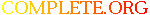Complete.Org: Mailing Lists: Archives: gopher: December 2003: [gopher] .names# [gopher] .names

[Top] [All Lists]

[Date Prev][Date Next][Thread Prev][Thread Next][Date Index] [Thread Index]
 To: gopher@xxxxxxxxxxxx Subject: [gopher] .names From: Chris Date: Tue, 16 Dec 2003 19:50:39 -0600 Reply-to: gopher@xxxxxxxxxxxx

```Hi guys,

Here is what I am using as .names now.
When run under gopherd 3.0 patch 5 it works properly
under pygopherd it doesnt.
What do you think...I have tried Numb in different places,
and trying just one file as opposed to all in the directory.
Actually Numb=1 IS working, the rest Name works but Numb doesnt.
Appreciate your help!!
Chris
(gopher://hal3000.cx)

####################################################################################################

Name= Welcome To The Hal3000 Gopher!
Type=i
Path=./test.txt
Numb=1

Name=Intro
Type=0
Path=./0index.txt
Numb=2

Name=News
Type=1
Path=./News
Numb=3

Name=Weather
Type=1
Path=./Weather
Numb=4

Name=Financial
Type=1
Path=./Financial
Numb=5

Name=Audio Visual
Type=1
Path=./Audio_Visual
Numb=6

Name=Software
Type=1
Path=./Software
Numb=7

Name=Recipes
Type=1
Path=./Recipes
Numb=8

Name=Phlog
Type=1
Path=./Phlog
Numb=9

Name=Misc
Type=1
Path=./Misc
Numb=10

Name=Links
Type=1
Path=./Links
Numb=11

#########################################################################################################

```

 [Prev in Thread] Current Thread [Next in Thread]